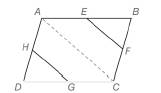Chapter 4.2, Problem 20E### Elementary Geometry for College St...

6th Edition
Daniel C. Alexander + 1 other
ISBN: 9781285195698

#### Solutions

Chapter
Section### Elementary Geometry for College St...

6th Edition
Daniel C. Alexander + 1 other
ISBN: 9781285195698
Textbook Problem
15 views

# In Exercises 19 to 24, complete each proof. Given: Quadrilateral A B C D , with midpoints E , F , G , and H of the sides Prove: E F ¯ ∥ H G ¯PROOF Statements Reasons 1. ? 1. Given 2. Draw A C ¯ 2. Through two points, there is one line 3. In ∆ A B C , E F ¯ ∥ A C ¯ and in ∆ A D C , H G ¯ ∥ A C ¯ 3. ? 4. ? 4. If two lines are ∥ to the same line, these lines are ∥ to each other

To determine

To complete:

The proof in the following table.

 PROOF Statements Reasons 1. ? 1. Given 2. Draw AC¯ 2. Through two points, there is one line 3. In ∆ABC, EF¯∥AC¯ and in ∆ADC, HG¯∥AC¯ 3. ? 4. ? 4. If two lines are ∥ to the same line, these lines are ∥ to each other
Explanation

Given,

Quadrilateral ABCD, with midpoints E, F, G, and H of the sides

Completion of the proof,

 PROOF Statements Reasons 1. Quadrilateral ABCD with midpoints E, F, G and H of the sides. 1. Given 2. Draw AC¯ 2. Through two points, there is one line 3. In ∆ABC, EF¯∥AC¯ and in ∆ADC, HG¯∥AC¯ 3. If a line segment divides two sides of a triangle proportionally, then the line segment is parallel to the third side of the triangle. 4. EF¯∥HG¯ 4. If two lines are ∥ to the same line, these lines are ∥ to each other

Final statement:

Therefore, the completed proof is,

 PROOF Statements Reasons 1

### Still sussing out bartleby?

Check out a sample textbook solution.

See a sample solution

#### The Solution to Your Study Problems

Bartleby provides explanations to thousands of textbook problems written by our experts, many with advanced degrees!

Get Started